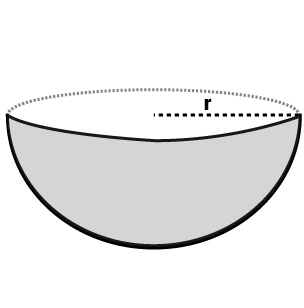# Surface Areas and Volume

Surface area and volume are calculated for any three-dimensional geometrical shape. The surface area of any given object is the area covered or region occupied by the surface of the object. Whereas volume is the amount of space available in an object. We have learned so far in geometry about different shapes and sizes such as sphere, cube, cuboid, cone, cylinder, etc. Each shape has its surface area as well as volume. But in the case of two-dimensional figures like square, circle, rectangle, triangle, etc., we can measure only the area covered by these figures and there is no volume available. Now, let us see the formulas of area and volume for different shapes.

## Area

The space occupied by a two-dimensional flat surface. It is measured in square units.

Generally, Area can be of two types

(i) Total Surface Area

(ii) Curved Surface Area

### Total surface area

Total surface area refers to the area including the base(s) and the curved part.

### Curved surface area (lateral surface area)

Refers to the area of only the curved part excluding its base(s).

## Volume

The amount of space, measured in cubic units, that an object or substance occupies. Some shapes are two-dimensional, so it doesn’t have volumes. Example, Volume of Circle cannot be found, though Volume of the sphere can be. It is so because a sphere is a three-dimensional shape.

Below given is the table for calculating Surface area and Volume for the basic geometrical figures:

 Name Perimeter Total Surface Area Curved Surface Area Volume Figure Square 4a a2 —- —-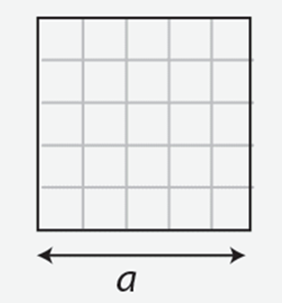Rectangle 2(w+h) w.h —- —-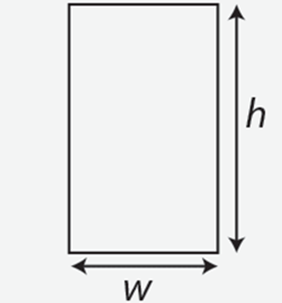Parallelogram 2(a+b) b.h —- —-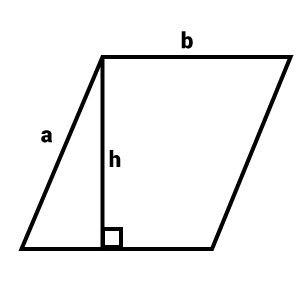Trapezoid a+b+c+d 1/2(a+b).h —- —-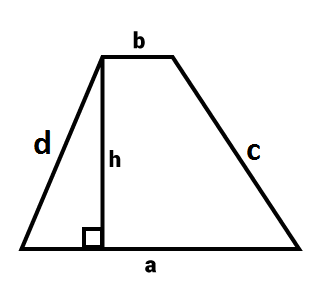Circle 2 π r π r2 —- —-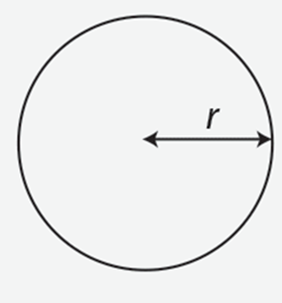Ellipse 2π√(a2 + b2)/2 π a.b —- —-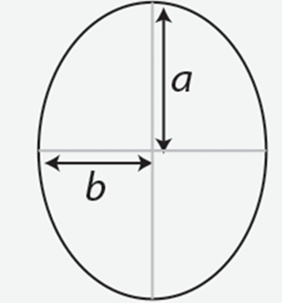Triangle a+b+c 1/2 * b * h —- —-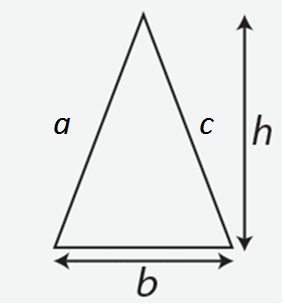Cuboid 4(l+b+h) 2(lb+bh+hl) 2h(l+b) l * b * h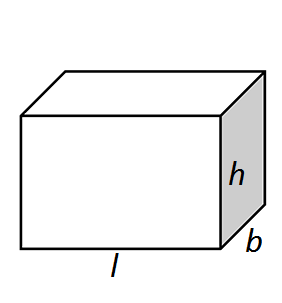Cube 6a 6a2 4a2 a3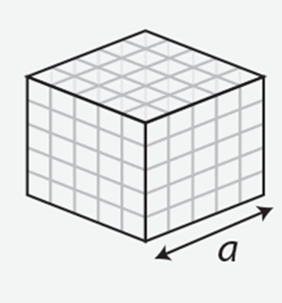Cylinder —- 2 π r(r+h) 2πrh π r2 h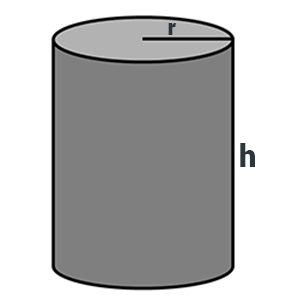Cone —- π r(r+l) π r l 1/3π r2 h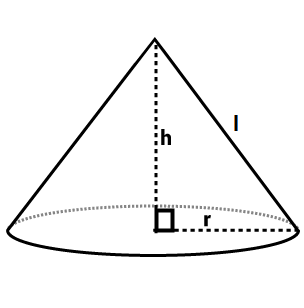Sphere —- 4 π r2 4π r2 4/3π r3Hemisphere —- 3 π r2 2 π r2 2/3π r3